A New Numerical Method for Solving Stiff Initial Value Problems | OMICS International
Fluid Mechanics: Open Access

# A New Numerical Method for Solving Stiff Initial Value Problems

B. Babangida*, H. Musa and L. K. Ibrahim

Department of Mathematics and Computer Sciences, Faculty of Natural and Applied Sciences, Umaru Musa Yar'adua University Katsina, Nigeria

*Corresponding Author:
B. Babangida
Department of Mathematics and Computer Sciences
Faculty of Natural and Applied Sciences
Katsina State, Nigeria
Tel: +2347067704150
E-mail: [email protected]

Received Date: September 25, 2016; Accepted Date: October 23, 2016; Published Date: October 30, 2016

Citation: Babangida B, Musa H, Ibrahim L. K. (2016) A New Numerical Method for Solving Stiff Initial Value Problems. Fluid Mech Open Acc 3: 136.

Copyright: © 2016 Babangida B, et al. This is an open-access article distributed under the terms of the Creative Commons Attribution License, which permits unrestricted use, distribution, and reproduction in any medium, provided the original author and source are credited.

Visit for more related articles at Fluid Mechanics: Open Access

#### Abstract

A new numerical method that computes 2–points simultaneously at each step of integration is derived. The numerical scheme is achieved by modifying an existing DI2BBDF method. The method is of order 2. The stability analysis of the new method indicates that it is both zero and A–stable, implying that it is suitable for stiff problems. The necessary and sufficient conditions for the convergence of the method are also established which proved the convergence of the method. Numerical results show that the method outperformed some existing algorithms in terms of accuracy.

#### Keywords

A-Stability; Order of a block method; Implicit block method; Stiff initial value problems; Convergence; Diagonally implicit; Zero stability

#### Introduction

Consider a system of first order stiff initial value problems (IVPs) of the form: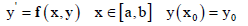(1)

System (1) can be regarded as stiff if its exact solution contains very fast as well as very slow components . Stiff IVPs occur in any fields of engineering and physical sciences. They are particularly found in electrical circuits, vibrations, chemical reactions, kinetics, automatic control and combustion, theory of fluid mechanics etc. The solution is characterized by the presence of transient and steady state components, which restrict the step size of many numerical methods except methods with A-stability properties (Suleiman [2,3]). This behaviour makes it difficult to develop suitable methods for solving stiff problems. However, efforts have been made by researchers, such as Abasi , Alt , Alvarez , Cash , Dahlquist , Ibrahim [8-10], Musa [11-14], Suleiman [2,3], Yatim  and Zawawi  among others, to develop methods for stiff ODEs. The need to obtain an efficient numerical approximation in terms of accuracy and computational time have attracted some researchers such as Alexander  with diagonally implicit Runge-Kutta for stiff ODEs, Ababneh  with design of new diagonally implicit Runge-Kutta for stiff problems, Ismail  with embedded pair of diagonally implicit Runge-Kutta for solving ODEs, Zawawi  with diagonally implicit block backward differentiation formulas for solving ODEs. The motivation of this research is to modify the method developed by Zawawi  so as to improve its accuracy and stability properties.

#### Derivation of the Method

This section describes the derivation of the method. Consider the numerical scheme developed by Zawawi .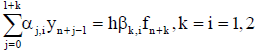(2)

To improve its accuracy and stability, the term -hβ(k,i) ρf(n+k-1) is added to (2) to come up with new scheme as follows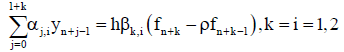(3)

Where, k=i=1 represents the first point, k=i=2 represents the second point and ρ ∈ (-1, 1). In this paper, the value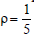is used. The formula (3) is derived from Taylor’s series expansion.

A Linear operator Li for the first and second point of the new method is defined by: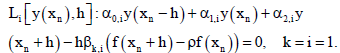(4)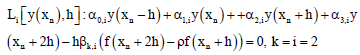(5)

respectively.

Expanding (4) and (5) as Taylor’s series about xn, collect like terms and normalized the coefficient of the first point α2,1 and second point α3,2 to obtain the following implicit 2–point block formula: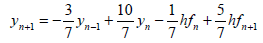(6)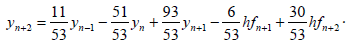The error constant of the new method (6) is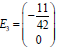implying that is of order 2.

Throughout this paper, the method will be referred to New Diagonally Implicit Super Class of Block Backward Differentiation Formula (NDISBBDF).

#### Stability Analysis of the NDISBBDF

This section presents the stability analysis of the method (6). It begins by presenting the definition of zero and A-stability taken from Suleiman .

Definition 3.1: A linear multistep method (LMM) is said to be zero stable if no root of the first characteristics polynomial has modulus greater than one and that any root with modulus one is simple.

Definition 3.2: A linear multistep method (LMM) is said to be A-stable if its stability region covers the entire negative half-plane.

Formula (6) can be written in matrix form as follows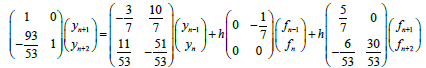(7)

Equation (7) can be rewritten in the following form: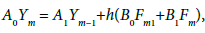(8)

Where,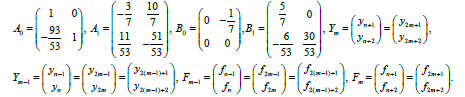Substituting the scalar test equation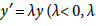is complex) into (8) and using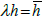gives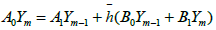(9)

The stability polynomial of (6) is obtained by evaluating: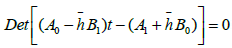(10)

to get,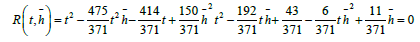(11)

To show that the method (6) is zero stable, we set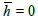in (11) to get the first characteristics polynomial as follows: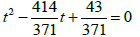(12)

Solving equation (12) for t gives the following roots:

t=0.1159 and t=1 (13)

From the definition 3.1, method (6) is zero-stable.

The boundary of the stability region of (6) is determined by substituting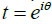into (11). The graph of stability region for (6) using maple is given in Figure 1.

The stability region covers the entire negative half plane indicating that the method (6) is A-stable.

#### Convergence of the Method

Convergence is an essential feature that every acceptable linear multistep method (LMM) must possess. This section discussed the convergence of the method (6). Consistency and zero stability are the necessary and sufficient conditions for the convergence of any numerical scheme. In section 3, it was shown that method (6) is zero stable. It is now remain to show that method (6) is consistent.

This discussion will be based on matrix form of (6) which can be written as: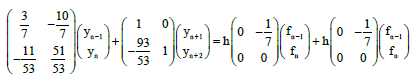(14)

With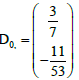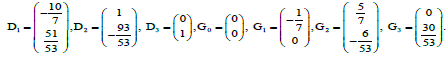Definition 4.1: Method (6) is consistent if and only if the following conditions are satisfied: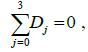(15)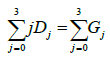(16)

Where, Dj,s and Gj,s are defined above.

Equation (15) and (16) then become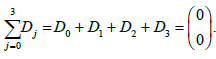(17)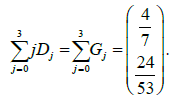(18)

Thus, the consistency conditions in (15) and (16) are therefore met. Hence, method (6) is consistent.

Since the method (6) is both consistent and zero stable, it is thus converges.

#### Implementation of the Method

This section discussed the implementation of the method using Newton iteration and begin by defining the absolute and maximum error.

Definition 5.1: Let yi and y(xi) be the approximate and exact solution of (1) respectively. Then the absolute error is given by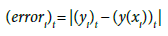(19)

The maximum error is given by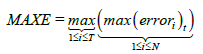(20)

Where, T is the total number of steps and N is the number of equations.

Define;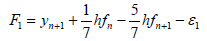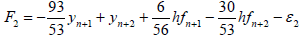Where,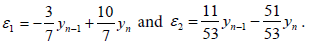Let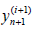denote the (i+1)th iteration and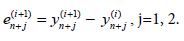(22)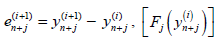(23)

This can be written in the form: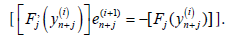(24)

Newton’s iteration for the new method takes the form: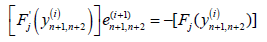(25)

In addition, in matrix form, equation (25) is equivalent to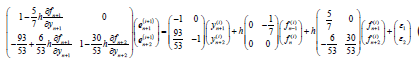(26)

#### Test Problems

The following problems are used to test the performance of the method.

Problem 1 (Musa )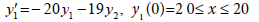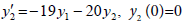Exact solution: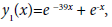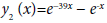Eigenvalues: -1 and -39.

Problem 2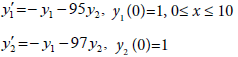Exact solution: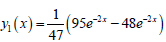,Eigenvalues: − 2 and − 96.

Problem 3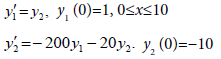Exact solution: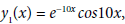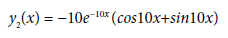NDISBBDF=New Diagonally Implicit Super Class of Block Backward Differentiation Formula

NS=Total Number of Steps

MAXE=Maximum Error

Time=Computational Time in Seconds

h=Step Size

To give the visual impact on the performance of the new method, the graphs of Log10 (MAXE) against h for the problems tested are plotted in figures 2-4.

#### Discussion

From tables 1-3, it can be seen that the new method outperformed the existing 2-point diagonally implicit block backward differentiation formula in terms of accuracy. Convergence is evident by the decrease in error as the step length h tends to zero. Similarly, the solution at any fixed point improves as the step length reduce. This can be seen from the tables when h is reduced (from 0.01, 0.001., 0.0001, and 0.00001 to 0.000001). The maximum error indicates that the numerical result becomes closer to the exact solution. Thus, the computed solution tends to the exact solution as the step length tends to zero. Hence, the new method converges faster for all the problems tested in comparison with DI2BBDF.

h Method NS MAXE Time
10-2 DI2BBDF
NDISBBDF
1000
1000
6.85453e-002
7.15278e-002
2.47400e-001
1.57000e-001
10-3 DI2BBDF
NDISBBDF
1000
1000
2.60436e-002
2.32062e-003
1.58700e-001
1.609000e-001
10-4 DI2BBDF
NDISBBDF
100000
100000
2.84730e-003
2.68751e-005
3.23700e-001
9.01200e-001
10-5 DI2BBDF
NDISBBDF
1000000
1000000
2.87174e-004
2.74330e-007
1.14900e+000
5.11600e+000
10-6 DI2BBDF
NDISBBDF
10000000
10000000
2.87419e-005
2.75064e-009
9.85100e+000
5.44700e+001

Table 1: Numerical result for problem 1.

h Method NS MAXE Time
10-2 DI2BBDF
NDISBBDF
500
500
9.37034e+005
2.22919e-002
2.51600e-001
1.75400e-001
10-3 DI2BBDF
NDISBBDF
5000
5000
5.58180e-002
1.20098e-002
2.43700e-001
1.331000e-001
10-4 DI2BBDF
NDISBBDF
50000
50000
7.04562e-003
1.61824e-004
2.61300e-001
3.05800e-001
10-5 DI2BBDF
NDISBBDF
500000
500000
7.19659e-004
1.69025e-006
7.92400e-001
2.3500e+000
10-6 DI2BBDF
NDISBBDF
5000000
5000000
7.21171e-005
1.70019e-008
5.05000e+000
2.58100e+001

Table 2: Numerical result for problem 2.

h Method NS MAXE Time
10-2 DI2BBDF
DIS2BBDF
500
500
1.61797e+000
1.63063e-001
1.17000e-001
1.19600e-001
10-3 DI2BBDF
DIS2BBDF
5000
5000
1.45914e-001
3.27724e-003
1.09700e-001
1.50200e-001
10-4 DI2BBDF
DIS2BBDF
50000
50000
1.44486e-002
3.56827e-005
2.22200e-001
3.46700e-001
10-5 DI2BBDF
DIS2BBDF
500000
500000
1.44346e-003
3.60983e-007
7.39800e-001
2.89700e+000
10-6 DI2BBDF
DIS2BBDF
5000000
5000000
1.44332e-004
3.61514e-009
5.54800e+000
2.559000e+001

Table 3: Numerical result for problem 3.

#### Numerical Results

The numerical results for the test problems given in section 6 are tabulated in this section. The problems are solved using the new method developed and the existing 2-point diagonally implicit block backward differentiation formula developed by Zawawi . The number of steps taken to complete the integration and the maximum error for the methods are presented and compared in Tables 1-3. In addition, the graph of Log10 (MAXE) against h for each problem is plotted. The notations used in the tables are listed below:

DI2BBDF=Diagonally Implicit 2-Point Block Backward Differentiation Formula

The graphs also show that the scaled errors for the new method are smaller when compared with that in the existing method.

#### Conclusion

A new method called New Diagonally Implicit Super Class of Block Backward Differentiation Formula (NDISBBDF) is developed. The order of the method is 2 and it is suitable for solving stiff IVPs. The stability analysis has shown that the method is both zero and A-stable. A comparison between the method and existing DI2BBDF is made and the results show that the method outperformed the existing DI2BBDF method in terms of accuracy.

#### References

Select your language of interest to view the total content in your interested language

### Article Usage

• Total views: 9809
• [From(publication date):
October-2016 - Dec 11, 2019]
• Breakdown by view type
• HTML page views : 9536Can't read the image? click here to refresh